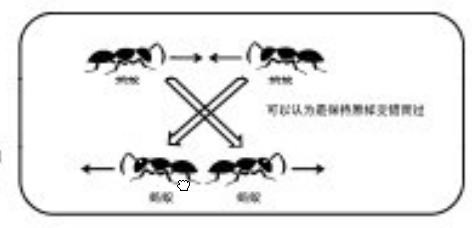# GitBook

Ants
 Time Limit: 1000MS Memory Limit: 30000K Total Submissions: 13088 Accepted: 5735

Description

An army of ants walk on a horizontal pole of length l cm, each with a constant speed of 1 cm/s. When a walking ant reaches an end of the pole, it immediatelly falls off it. When two ants meet they turn back and start walking in opposite directions. We know the original positions of ants on the pole, unfortunately, we do not know the directions in which the ants are walking. Your task is to compute the earliest and the latest possible times needed for all ants to fall off the pole.

Input

The first line of input contains one integer giving the number of cases that follow. The data for each case start with two integer numbers: the length of the pole (in cm) and n, the number of ants residing on the pole. These two numbers are followed by n integers giving the position of each ant on the pole as the distance measured from the left end of the pole, in no particular order. All input integers are not bigger than 1000000 and they are separated by whitespace.

Output

For each case of input, output two numbers separated by a single space. The first number is the earliest possible time when all ants fall off the pole (if the directions of their walks are chosen appropriately) and the second number is the latest possible such time.

Sample Input

```2
10 3
2 6 7
214 7
11 12 7 13 176 23 191
```

Sample Output

```4 8
38 207```

n只蚂蚁以每秒1cm的速度在长为Lcm的竿子上爬行。当蚂蚁爬到竿子的端点时就会掉落。由于竿子太细，两只蚂蚁相遇时，它们不能交错通过，只能各自反向爬回去。对于每只蚂蚁，我们知道它距离竿子左端的距离xi，但不知道它当前的朝向。请计算所有蚂蚁落下竿子所需的最短时间和最长时间。(果然一个木桶能装多少水取决于最短的那个木板嘛)

```#include<cstdio>
#include<algorithm>
using namespace std;
int a;
int main() {
int T,L,n,Tmin,Tmax;
scanf("%d",&T);
while(T--) {
scanf("%d %d",&L,&n);
for(int i=0; i<n; i++)
scanf("%d",&a[i]);
Tmin=Tmax=0;
for(int i=0; i<n; i++)
Tmin=max(Tmin,min(a[i],L-a[i]));
for(int i=0; i<n; i++)
Tmax=max(Tmax,max(a[i],L-a[i]));
printf("%d %d\n",Tmin,Tmax);
}
return 0;
}```

-.-